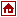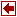## Nonlinear Programming Packages

 MINQ Author Prof. Dr. Arnold Neumaier Institut für Mathematik Universität Wien Strudlhofgasse 4 A-1090 Wien Austria Office: Sensengasse 8/16 Phone:+431 407 6363-2 Fax: +431 407 6363-1 (or +431 31367 4040) Email: neum@cma.univie.ac.at Language Matlab Algorithm The method consists of a combination of coordinate searches and subspace minimization steps, suitable for both dense and sparse matrices. (In the sparse case, it is assumed that the variables are already ordered such that the symbolic factorization of G is sparse.) Input Format Modeling Languages link Commercial Status free Platform Remarks MINQ is a Matlab program for bound constrained indefinite quadratic programming based on 7rank 1 modifications. It solves the optimization problem min fct = c^T x + 0.5 x^T G x s.t. x in [xu,xo] (componentwise) where G is a symmetric n x n matrix, not necessarily semidefinite, and infinite bounds are allowed. MINQ can also be used for general definite quadratic programming since the dual is simply constrained. Based on this, the Matlab program MINQDEF solves the optimization problem min fct = c^T x + 0.5 x^T G x s.t. A x >= b, with equality at indices with eq=1 where G is a positive definite symmetric n x n matrix, and the Matlab program MINQSEP solves the same problem with definite diagonal G. (In the sparse case, it is now assumed that the variables are already ordered such that the symbolic factorizations of G and AG^(-1)A^T are sparse.) The required m-files can be downloaded as the gzipped tar file minq.tar.gz (8K). References